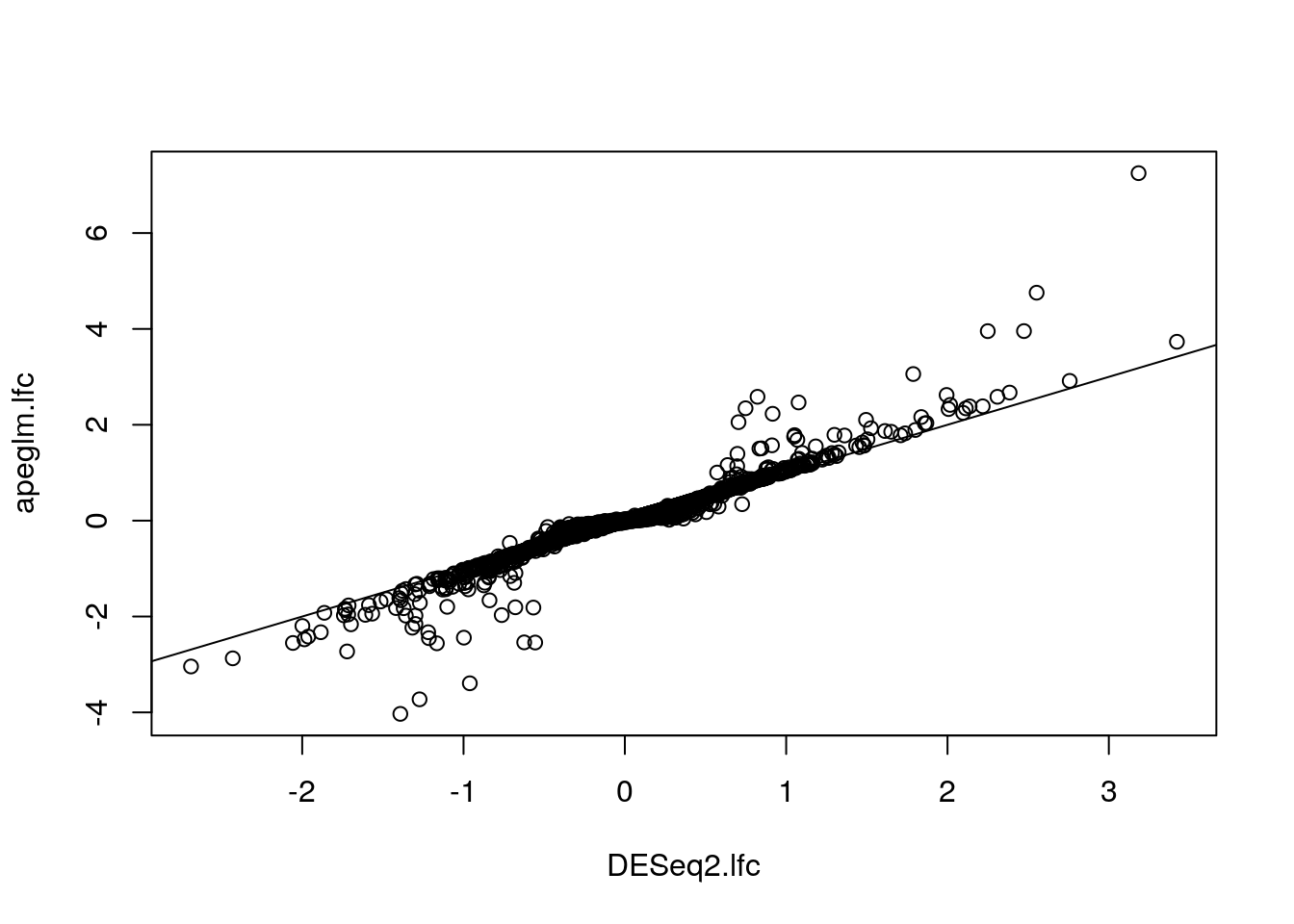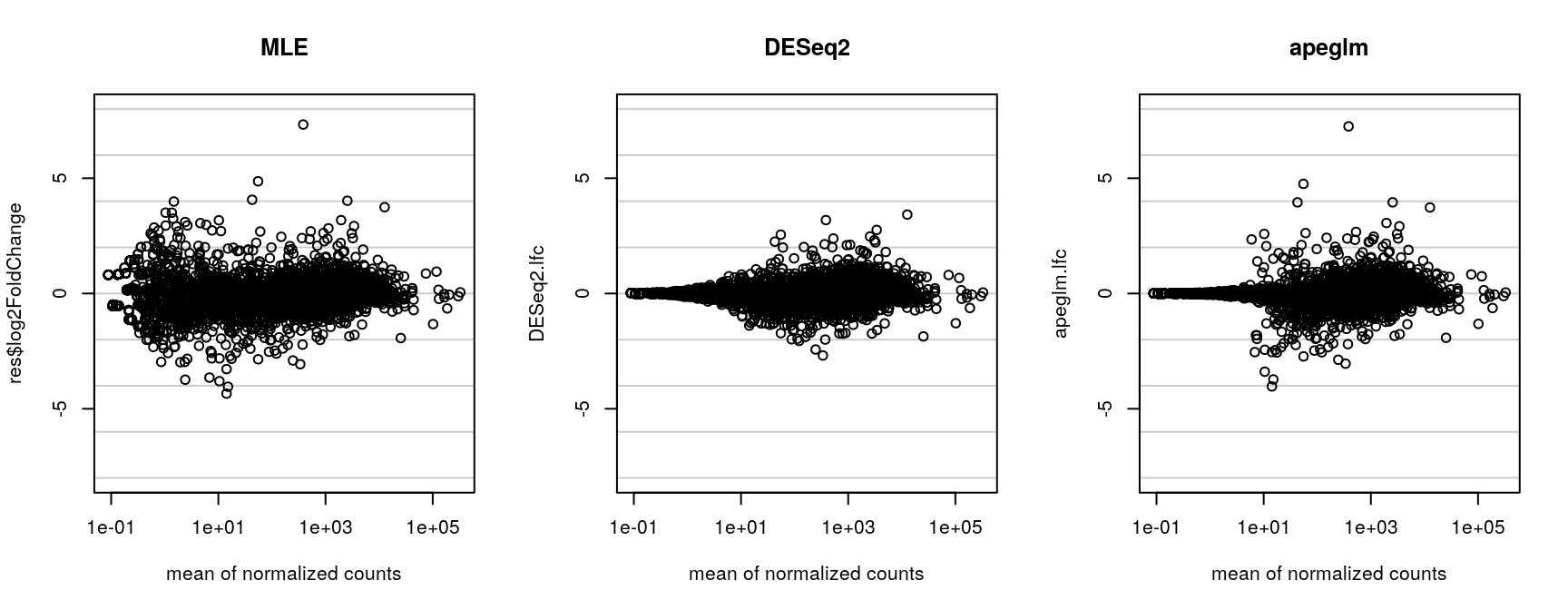# Typical RNA-seq call from DESeq2

Note: the typical RNA-seq workflow for users would be to call apeglm estimation from within the `lfcShrink` function from the DESeq2 package. The unevaluated code chunk shows how to obtain apeglm shrinkage estimates after running `DESeq`. See the DESeq2 vignette for more details. The `lfcShrink` wrapper function takes care of many details below, and unifies the interface for multiple shrinkage estimators. The coefficient to shrink can be specified either by name or by number (following the order in `resultsNames(dds)`). Be aware that DESeq2’s `lfcShrink` interface provides LFCs on the log2 scale, while apeglm provides coefficients on the natural log scale.

``res <- lfcShrink(dds, coef=2, type="apeglm")``

# Acknowledgments

Joshua Zitovsky contributed fast C++ code for the beta-binomial likelihood, demonstrated in a later section of the vignette.

We have benefited in the development of `apeglm` from feedback or contributions from the following individuals:

Wolfgang Huber, Cecile Le Sueur, Charlotte Soneson

# Example RNA-seq analysis

Here we show example code which mimics what will happen inside the `lfcShrink` function when using the apeglm method (Zhu, Ibrahim, and Love 2018).

Load a prepared `SummarizedExperiment`:

``````library(airway)
data(airway)
``````##                 SRR1039508 SRR1039509 SRR1039512 SRR1039513 SRR1039516
## ENSG00000000003        679        448        873        408       1138
## ENSG00000000005          0          0          0          0          0
## ENSG00000000419        467        515        621        365        587
## ENSG00000000457        260        211        263        164        245
## ENSG00000000460         60         55         40         35         78
## ENSG00000000938          0          0          2          0          1
##                 SRR1039517 SRR1039520 SRR1039521
## ENSG00000000003       1047        770        572
## ENSG00000000005          0          0          0
## ENSG00000000419        799        417        508
## ENSG00000000457        331        233        229
## ENSG00000000460         63         76         60
## ENSG00000000938          0          0          0``````

For demonstration, we will use 5000 genes of the `airway` dataset, the first from those genes with at least 10 counts across all samples.

``````keep <- head(which(rowSums(assay(airway)) > 0), 5000)
airway <- airway[keep,]``````

First run a DESeq2 differential expression analysis (Love, Huber, and Anders 2014) (size factors, and dispersion estimates could similarly be estimated using edgeR):

``````library(DESeq2)
dds <- DESeqDataSet(airway, ~cell + dex)
dds\$dex <- relevel(dds\$dex, "untrt")
dds <- DESeq(dds)
res <- results(dds)``````

Defining data and parameter objects necessary for `apeglm`. We must multiply the coefficients from DESeq2 by a factor, because apeglm provides natural log coefficients. Again, this would be handled inside of `lfcShrink` in DESeq2 for a typical RNA-seq analysis.

``````x <- model.matrix(design(dds), colData(dds))
param <- dispersions(dds)
mle <- log(2) * cbind(res\$log2FoldChange, res\$lfcSE)
offset <- matrix(log(sizeFactors(dds)),
ncol=ncol(dds),
nrow=nrow(dds),byrow=TRUE)``````

# Running apeglm

Here `apeglm` on 5000 genes takes less than a minute on a laptop. It scales with number of genes, the number of samples and the number of variables in the design formula, where here we have 5 coefficients (one for the four cell cultures and one for the difference due to dexamethasone treatment).

We provide `apeglm` with the SummarizedExperiment although the function can also run on a matrix of counts or other observed data. We specify a `coef` as well as a `threshold` which we discuss below. Note that we multiple the `threshold` by `log(2)` to convert from log2 scale to natural log scale.

``````library(apeglm)
system.time({
fit <- apeglm(Y=airway, x=x, log.lik=logLikNB, param=param, coef=ncol(x),
threshold=log(2) * 1, mle=mle, offset=offset)
})``````
``````##    user  system elapsed
##  26.847   0.000  26.847``````
``str(fit\$prior.control)``
``````## List of 7
##  \$ no.shrink            : int [1:4] 1 2 3 4
##  \$ prior.mean           : num 0
##  \$ prior.scale          : num 0.299
##  \$ prior.df             : num 1
##  \$ prior.no.shrink.mean : num 0
##  \$ prior.no.shrink.scale: num 15
##  \$ prior.var            : num 0.0894``````

There are faster implementations of apeglm specifically for negative binomial likelihoods. The version `nbinomR` is ~5 times faster than the default `general`.

``````system.time({
fitR <- apeglm(Y=assay(airway), x=x, log.lik=NULL, param=param, coef=ncol(x),
threshold=log(2) * 1, mle=mle, offset=offset, method="nbinomR")
})``````
``````##    user  system elapsed
##   6.168   0.000   6.168``````

The version `nbinomCR` is ~10 times faster than the default `general`.

``````system.time({
fitCR <- apeglm(Y=assay(airway), x=x, log.lik=NULL, param=param, coef=ncol(x),
threshold=log(2) * 1, mle=mle, offset=offset, method="nbinomCR")
})``````
``````##    user  system elapsed
##   3.172   0.000   3.172``````

The version `nbinomC` returns only the MAP coefficients and can be ~50-100 times faster than the default `general`. The MAP coefficients are the same as returned by `nbinomCR` above, we just skip the calculation of posterior SD. A variant of `nbinomC` is `nbinomC*` which includes random starts.

``````system.time({
fitC <- apeglm(Y=assay(airway), x=x, log.lik=NULL, param=param, coef=ncol(x),
threshold=log(2) * 1, mle=mle, offset=offset, method="nbinomC")
})``````
``````##    user  system elapsed
##   0.202   0.000   0.202``````

Among other output, we have the estimated coefficients attached to the ranges of the SummarizedExperiment used as input:

``class(fit\$ranges)``
``````##  "CompressedGRangesList"
## attr(,"package")
##  "GenomicRanges"``````
``mcols(fit\$ranges, use.names=TRUE)``
``````## DataFrame with 5000 rows and 5 columns
##                       X.Intercept.         cellN061011         cellN080611
##                          <numeric>           <numeric>           <numeric>
## ENSG00000000003   6.64565685883073  0.0607881068431503   0.229672330993784
## ENSG00000000419   6.23452224381349 -0.0849990629089554 -0.0151414731603562
## ENSG00000000457   5.45295046300281   0.060167029799732 -0.0556310344711023
## ENSG00000000460   3.77047988584564   0.545203896907685   0.261356100292042
## ENSG00000000938 -0.314689550020255   -4.17748981922556  -0.539678670121058
## ...                            ...                 ...                 ...
## ENSG00000122756  -3.62985171791685    4.03910910287823   -2.00591343214784
## ENSG00000122778   5.23905962019427  0.0800614479130158   0.785718241416305
## ENSG00000122779   5.66860926529674 -0.0569747440557475  0.0501292974551331
## ENSG00000122783   6.01534721390228  0.0368918236864347  -0.157828298844619
## ENSG00000122786   9.78251386175223  -0.182349284400113  0.0942307382696345
##                          cellN61311                dextrt
##                           <numeric>             <numeric>
## ENSG00000000003  -0.152039407731112     -0.26470148486435
## ENSG00000000419 -0.0476622243520274     0.115132140588663
## ENSG00000000457   0.048462268853839   0.00907000359520414
## ENSG00000000460   0.349429848485865   -0.0425238103188782
## ENSG00000000938   -4.20084641398391  -0.00836574242438389
## ...                             ...                   ...
## ENSG00000122756    2.95799390247506 -0.000701743021366193
## ENSG00000122778   0.240098604814333    -0.285457153643358
## ENSG00000122779 -0.0441315171480504    -0.109945276881165
## ENSG00000122783  -0.297399861535955    0.0690120376614942
## ENSG00000122786  -0.120280028800808     0.523514860322633``````

We can compare the coefficients from apeglm with those from DESeq2. apeglm provides coefficients on the natural log scale, so we must convert to log2 scale by multiplying by `log2(exp(1))`. Note that DESeq2’s `lfcShrink` function converts apeglm coefficients to the log2 scale internally.

``````system.time({
res.shr <- lfcShrink(dds, coef=5)
})``````
``````##    user  system elapsed
##   2.363   0.024   2.387``````
``````DESeq2.lfc <- res.shr\$log2FoldChange
apeglm.lfc <- log2(exp(1)) * fit\$map[,5]``````

Here we plot apeglm estimators against DESeq2:

``````plot(DESeq2.lfc, apeglm.lfc)
abline(0,1)``````Here we plot MLE, DESeq2 and apeglm estimators against the mean of normalized counts:

``````par(mfrow=c(1,3))
lims <- c(-8,8)
hline <- function() abline(h=c(-4:4 * 2),col=rgb(0,0,0,.2))
xlab <- "mean of normalized counts"
plot(res\$baseMean, res\$log2FoldChange, log="x",
ylim=lims, main="MLE", xlab=xlab)
hline()
plot(res\$baseMean, DESeq2.lfc, log="x",
ylim=lims, main="DESeq2", xlab=xlab)
hline()
plot(res\$baseMean, apeglm.lfc, log="x",
ylim=lims, main="apeglm", xlab=xlab)
hline()``````# Specific coefficients

Note that p-values and FSR define different events, and are not on the same scale. An FSR of 0.5 means that the estimated sign is as bad as random guess.

``````par(mfrow=c(1,2),mar=c(5,5,1,1))
plot(res\$pvalue, fit\$fsr, col="blue",
xlab="DESeq2 pvalue", ylab="apeglm local FSR",
xlim=c(0,1), ylim=c(0,.5))
abline(0,1)
plot(-log10(res\$pvalue), -log10(fit\$fsr),
xlab="-log10 DESeq2 pvalue", ylab="-log10 apeglm local FSR",
col="blue")
abline(0,1)``````